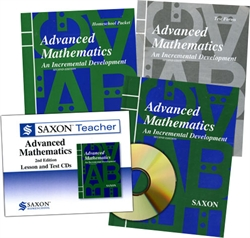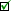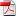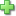# Saxon Advanced Mathematics - Home School Bundle with Teacher CD

Publisher: Saxon Publishers
2nd Edition, Item: 33116
Curriculum Bundle
List Price: \$229.80 Our Price: \$206.83
Used Price: \$160.86 (1 in stock)Condition Policy

See series description for full review.

In Advanced Mathematics, topics from advanced algebra, geometry, trigonometry, discrete mathematics, and mathematical analysis are interwoven to form a fully integrated text. Specific topics include permutations and combinations; trigonometric identities; inverse trigonometric functions; conic sections; graphs of sinusoids; rectangular and polar representation of complex numbers; De Movie's theorem; matrices and determinants; the binomial theorem; and the rational roots theorem. Euclidean geometry is also included. (125 lessons)

The Home Study Kit, which contains the student textbook, answer key and test packet, and the Saxon Teacher CD (which contains lecture CDs and the complete solutions) are both included in this bundle. You will need a scientific calculator, but since there are optional exercises for a graphing calculator, that would be even better.

## Samples:Table of ContentsLesson 2 page 1Lesson 2 page 2Test 7Answer KeyClick here to write a review